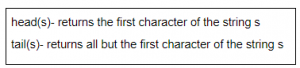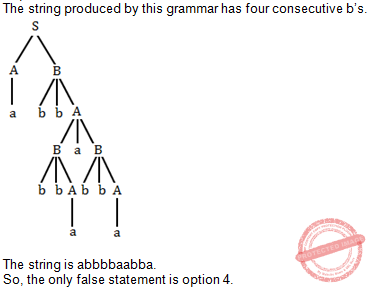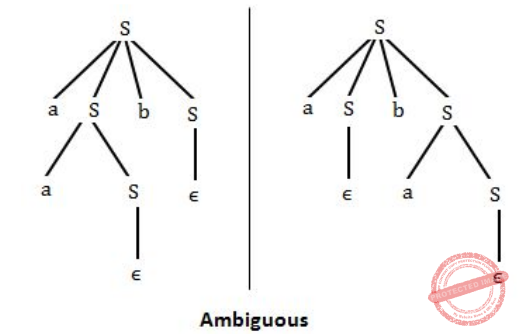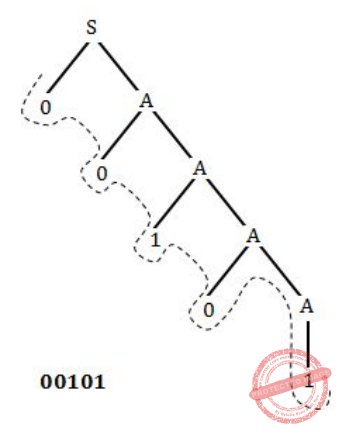## Languages-and-Grammars

 Question 1
A language with string manipulation facilities uses the following operations.concat(sl, s2)- concatenates string s1 with s2. The output of concat(head(s), head(tail(tail(s)))), where s is acbc is
 A ab B ba C ac D as
Theory-of-Computation       Languages-and-Grammars       ISRO-2018
Question 1 Explanation:Question 2
Given the following statements:
S1 : Every context-sensitive language L is recursive
S2 : There exists a recursive language that is not context-sensitive
Which statements are true?
 A Only S1 is correct B Only S2 is correct C Both S1 and S2 are not correct D Both S1 and S2 are correct
Theory-of-Computation       Languages-and-Grammars       ISRO-2017 May
Question 2 Explanation:
According to the Chomsky hierarchy, both the statements are correct.Question 3
Identify the language generated by the following grammar
S -> AB
A -> aAb|ϵ
B -> bB| b
 A {ambn | n>=m, m>0} B {ambn | n>=m, m>=0} C {ambn | n>m, m>0} D {ambn | n>m, m>=0}
Theory-of-Computation       Languages-and-Grammars       ISRO-2017 December
Question 3 Explanation:
The language generated by given grammar is - L={b,bb,abb,abbb,ababb}
Hence it is ambn | n>m, m>=0
 Question 4
S → aSa ∣ bSb ∣ a ∣ b The language generated by the above grammar over the alphabet is the set of
 A all palindromes B all odd length palindromes C strings that begin and end with the same symbol D all even length palindromes
Theory-of-Computation       Languages-and-Grammars       ISRO-2016
Question 4 Explanation:
From the grammar, we can easily infer that it generates all the palindromes of odd length, for ex: strings {aba, bab, aaa, bbb, ….}
 Question 5
What is the highest type number that can be assigned to the following grammar?
S → Aa
A → Ba
B → abc
 A Type 0 B Type 1 C Type 2 D Type 3
Theory-of-Computation       Languages-and-Grammars       ISRO-2016
Question 5 Explanation:
Grammar is belongs to Type 0 ,Type 1,Type 2,Type 3 because it is regular. Option D is right answer because they are asking only highest type number.Question 6
What is the number of steps required to derive the string ((() ()) ()) for the following grammar?
S → SS
S → (S)
S → ε
 A 10 B 15 C 12 D 16
Theory-of-Computation       Languages-and-Grammars       ISRO CS 2014
Question 6 Explanation:
The required string consists of only only matched parenthesis enclosed with parentheses.
To generate the string ((() ()) ()) , we need to perform the steps sequentially.
As the string starts with left parentheses, we will start with the production S → (S).
Depending upon the string we will go for either left or right tree generation.
1) S → (S) // Replacing S by (S)
2) S → (SS) // Replacing by S by SS
3) S → ((S)S) //Replacing S by(S)
4) S → ((SS)S)// Replacing S by SS
5) S → (((S)S))S)
6) S → (((S)(S))S)
7) S → (((S)(S))(S)) [S → ε]
8) S → ((()(S))S) [S → ε]
9) S → ((()())S) [S → ε]
10) S → ((()()))
 Question 7
Consider the following grammar.
S → AB
A → a
A → BaB
B → bbA
Which of the following statements is FALSE?
 A The length of every string produced by this grammar is even B No string produced by this grammar has three consecutive a’s C The length of substring produced by B is always odd D No string produced by this grammar has four consecutive b’s
Theory-of-Computation       Languages-and-Grammars       ISRO CS 2014
Question 7 Explanation:Question 8
The number of substrings that can be formed from string given by “a d e f b g h n m p” is
 A 10 B 45 C 56 D 55
Theory-of-Computation       Languages-and-Grammars       UGC NET CS 2018-DEC Paper-2
Question 8 Explanation:
If we have no repetition in a string then the number of substrings can be found using the formula :
n*(n+1)/2 + 1
We have added 1 because it may include a NULL string also.
The number of substrings = 10*(11)/2 +1
The number of substrings = 56
 Question 9
Which of the following correctly defines kleene closure?
 A It is the infinite set of all possible strings of all possible lengths over Σ(input set) excluding ʎ(empty string) B It is the finite set of all possible strings of all possible lengths over Σ(input set) excluding ʎ(empty string) C It is the finite set of all possible strings of all possible lengths over Σ(input set) D It is the infinite set of all possible strings of all possible lengths over Σ(input set)
Theory-of-Computation       Languages-and-Grammars       JT(IT) 2018 PART-B Computer Science
Question 9 Explanation:
Kleene closure
It is the infinite set of all possible strings of all possible lengths over Σ(input set) excluding ʎ(empty string).
 Question 10

A language is a subset of Σ* for some alphabet Σ. If a language takes all possible strings of length 2 over Σ = {a,b}, then, which of the below is correct?

 A L = {ab,bb,ba} B L = {aa,ab,ba,bb} C L = {ab,bb,aa} D L = {ab,ba,aa}
Theory-of-Computation       Languages-and-Grammars       JT(IT) 2018 PART-B Computer Science
Question 10 Explanation:
→ All possible strings of length 2 over Σ = {a,b} = 4(aa,ab,ba,bb}.
→ We are computing all possible strings using 2n formula. Where n is number of strings.
 Question 11
Which of the definitions generates the same languages as L, where
L={ x​n​ y​n​ , n>=1 }
i. E→ xEy |xy
ii.xy|x​ +​ x yy​ +
iii. x​ +​ y​ +
 A i only B i and ii only C ii and iii only D ii only
Theory-of-Computation       Languages-and-Grammars       Nielit Scientific Assistance IT 15-10-2017
Question 11 Explanation:
The language L={ x​ n​ y​ n​ , n>=1 }
For n=1, xy
n=2,x​ 2​ y​ 2
n=3,x​ 3​ y​ 3
In the above language , there are equal number of x’s followed by y’s
Definition-1:E→ xEy |xy → xEy → xxEyy → xxxEyyy → xxxxyyyy
Which is nothing but equal number of x’s followed by equal number of y’s
X​ +​ means one or more x’s
Y​ +​ means one or more y’s
Definition -2 :
The expression xy|x​ +​ x yy​ + ​ gives xy, xxyy , xxxxyy , xxyyyyy and so on
The definition-2 won’t give equal number of x’s followed by y’s
It generates any number of x’s followed by any number of y’s.
Definition -3 :
The expression x​ +​ y​ +​ gives xy, xxy,xxxy,xyy,xyyy and so on strings.
The definition-3 won’t give equal number of x’s followed by y’s
It generates any number of x’s followed by any number of y’s.
 Question 12
Which of the definitions generates the same languages as L, where
L={ x​ n​ y​ n​ , n>=1 }
i. E→ xEy |xy
ii.xy|x​ +​ x yy​ +
iii. x​ +​ y​ +
 A i only B i and ii only C ii and iii only D ii only
Theory-of-Computation       Languages-and-Grammars       Nielit Scientific Assistance CS 15-10-2017
Question 12 Explanation:
The language L={ x​ n​ y​ n​ , n>=1 }
For n=1, xy
n=2,x​ 2​ y​ 2
n=3,x​ 3​ y​ 3
In the above language , there are equal number of x’s followed by y’s
Definition-1​ :E→ xEy |xy → xEy → xxEyy → xxxEyyy → xxxxyyyy
Which is nothing but equal number of x’s followed by equal number of y’s
X​
+​
means one or more x’s
Y​
+
​ means one or more y’s
Definition -2 : The expression xy|x​ +​ x yy​ + ​ gives xy, xxyy , xxxxyy , xxyyyyy and so on
The definition-2 won’t give equal number of x’s followed by y’s
It generates any number of x’s followed by any number of y’s.
Definition -3 :
The expression x​ +​ y​ +​ gives xy, xxy,xxxy,xyy,xyyy and so on strings.
The definition-3 won’t give equal number of x’s followed by y’s
It generates any number of x’s followed by any number of y’s.
 Question 13

For the context free grammar G:

```R →  XRX|S,
S →  aTbTa,
T →  XTX|Xϵ
X → a|b```

The strings which are not in L(G) are:

 A ab,ba,aab B abb,aabab C a,b,aa D a,b,ϵ
Theory-of-Computation       Languages-and-Grammars       JT(IT) 2016 PART-B Computer Science
Question 13 Explanation:
We can’t generate the strings “a” and “b” because the Starting symbol is R→ XRX|S.
 Question 14
Which of the following is FALSE?
 A The grammar S→aS|aSbS|∈, where S is the only non-terminal symbol, and ∈ is the null string, is ambiguous. B An unambiguous grammar has same leftmost and rightmost derivation. C An ambiguous grammar can never be LR(k) for any k. D Recursive descent parser is a top-down parser.
Theory-of-Computation       Languages-and-Grammars       UGC NET CS 2016 Aug- paper-2
Question 14 Explanation:
Option-A:​ TRUE: S is non terminal symbol, ∈ is the null string, is ambiguous.Option-B:​ FALSE: An unambiguous grammar has same leftmost and rightmost derivation.
Option-C:​ TRUE: An ambiguous grammar can never be LR (k) for any k, because LR(k) algorithm aren’t designed to handle ambiguous grammars. It would get stuck into undecidability problem, if employed upon an ambiguous grammar, no matter how large the constant k is.
Option-D: ​ TRUE: Recursive descent parser is a top-down parser
 Question 15
Which sentence can be generated by
S→d/bA
A→d/ccA
 A bccddd B aabccd C ababccd D abbbd E None of the above
Theory-of-Computation       Languages-and-Grammars       UGC NET CS 2005 Dec-Paper-2
Question 15 Explanation:
Here, there is no terminal symbol ‘a’, so the answer never be option B,C,D.
Option-A is also wrong because the string generated by grammar is bccccd.Question 16
Which of the following is the most general phase-structured grammar ?
 A Regular B Context-sensitive C Context free D Syntax tree
Theory-of-Computation       Languages-and-Grammars       UGC NET CS 2006 Dec-paper-2
Question 16 Explanation:
→ Phrase structure grammars are also known as constituency grammars. The defining trait of phrase structure grammars is thus their adherence to the constituency relation, as opposed to the dependency relation of dependency grammars.
→ Most general phase structured grammar is context sensitive grammar.
 Question 17
Which of the following strings is in the language defined by grammar
S → 0A
A → 1A/0A/1
 A 01100 B 00101 C 10011 D 11111
Theory-of-Computation       Languages-and-Grammars       UGC NET CS 2006 June-Paper-2
Question 17 Explanation:Question 18
LL grammar for the language L = {an bm cn+m | m≥0, n≥0} is
 A S → aSc | S1 ; S1 → bS1c | λ B S → aSc | S1| λ ; S1 → bS1c C S → aSc | S1| λ ; S1 → bS1c| λ D S → aSc | λ ; S1 → bS1c| λ|
Theory-of-Computation       Languages-and-Grammars       UGC NET CS 2013 Sep-paper-2
Question 18 Explanation:
L1 is the language when n=0, m=0.
L1={λ}
L2 is the language when only n=0
L2={bc, bbcc, bbbccc,......}
L3 is the language when only m=0
L3={ ac, aacc, aaaccc, ........}
L4 is the language when n≠0 and m≠0
L4= {abcc, aabbcccc, aaabbbcccccc,...............}
L= {L1 U L2 U L3 U L4}
L= { λ, bc, bbcc, bbbccc,........, ac, aacc, aaaccc,.........., abcc, aabbcccc,aaabbbcccccc,.........}
Option A is incorrect because it does not have any stoping point for condition when m=0 i.e it can't generate strings { ac, aacc, aaaccc, ........}
Option B is incorrect because it does not have any stopping point for condition when n=0 i.e it can't generate strings {bc, bbcc, bbbccc,......}
Option C is correct because it can generate all the strings generated by the language L.
Option D is incorrect because state S1 is unreachable in it. And because of this strings {bc, bbcc, bbbccc,......{abcc, aabbcccc, aaabbbcccccc,...............} can't be generated.
 Question 19
The ‘C’ language is
 A Context free language B Context sensitive language C Regular language D None of the above
Theory-of-Computation       Languages-and-Grammars       UGC NET CS 2012 Dec-Paper-2
Question 19 Explanation:
C and C++ are context sensitive languages.
 Question 20
The context free grammar for the language L = {an bm | n ≤ m + 3, n ≥ 0, m ≥ 0} is
 A S → aaa A; A → aAb | B, B → Bb | λ B S → aaaA|λ, A → aAb | B, B → Bb | λ C S → aaaA | aa A | λ, A → aAb | B, B → Bb| λ D S → aaaA | aa A | aA | λ, A → aAb | B, B → Bb | λ
Theory-of-Computation       Languages-and-Grammars       UGC NET CS 2013 Dec-paper-2
Question 20 Explanation:
According to given the given language L when
m=0, n<= 3 i.e. L1= { λ, a, aa, aaa}
m=1, n<=4 i.e. L2={ ab, aab, aaab, aaaab}
m=2, n<=5 i.e. L3={abb, aabb, aaabb, aaaabb, aaaaabb} and so on.
Hence L = L1 U L2 U L3 U ...........
L= { λ, a, aa, aaa, ab, aab, aaab, aaaab, abb, aabb, aaabb, aaaabb, aaaabb, .................}
Option(A) is not correct because it can't generate λ.
Option(B) is not correct because it can't generate "a".
Option(C) is not correct because it can't generate "a".
Option(D) is correct because it is generating the language L
.
 Question 21
Which of the following is the most general phase structured grammar ?
 A Regular B Context-sensitive C Context free D None of the above
Theory-of-Computation       Languages-and-Grammars       UGC NET CS 2010 June-Paper-2
Question 21 Explanation:
→ Phrase structure grammars are also known as constituency grammars. The defining trait of phrase structure grammars is thus their adherence to the constituency relation, as opposed to the dependency relation of dependency grammars.
→ Most general phase structured grammar is context sensitive grammar.
 Question 22
The language L = {ai b ci │ i >= 0} over the alphabet {a, b, c} is:
 A a regular language. B not a deterministic context free language but a context free language. C recursive and is a deterministic context free language. D not recursive.
Theory-of-Computation       Languages-and-Grammars       UGC NET CS 2017 Nov- paper-3
Question 22 Explanation:
→ The given language is Context free language(CFL) because we can push a's onto the top of stack as a's come as input and when a "b" come as input don't push it on the top of stack just change the state and after that when c's come come as input pop one "a" from top of stack for each "c".
→ In this way the given language can be accepted by the deterministic pushdown automata and hence the language is deterministic context free language. And when a language is CFL it is also a recursive language.
Hence the correct answer is option (C)
 Question 23
Consider the languages L1 = φ and L2 = {1}. Which one of the following represents L*1∪ L*1 L*2 ?
 A {∈} B {∈, 1} C ϕ D 1*
Theory-of-Computation       Languages-and-Grammars       UGC NET CS 2017 Jan- paper-3
Question 23 Explanation:
As we know, for any regular expression R,
Rϕ = ϕR=ϕ
So L1 L2 * = ϕ
and L1 * = {ϕ}* = {ϵ}
So L1L2* ∪ L1* = {ϵ}
 Question 24
Which of the following statements is false?
 A Every context-sensitive language is recursive. B The set of all languages that are not recursively enumerable is countable. C The family of recursively enumerable languages is closed under union. D The families of recursively enumerable and recursive languages are closed under reversal.
Theory-of-Computation       Languages-and-Grammars       UGC NET CS 2017 Jan- paper-3
Question 24 Explanation:
Option(A) : it is correct option because L= {an bn cn} is a context sensitive language and it can also be accepted by the turing machine. Hence every CSL also recursive language.

Option(B): It is incorrect option.

Option(C): Yes it is true that recursively enumerable languages are closed under union.

Option(D): It is a correct option because recursively enumerable and recursive languages are closed under reversal.

NOTE: The difference between recursive and recursively enumerable language is that if a language is recursive then there will be definitely a turing machine which will halt and will say whether the string is accepted or not. But if a language is recursively enumerable then the turing machine will halt if the string is accepted but it is not necessary that the turing machine will halt to indicate that the string is not accepted. in this case the user might end up in infinite wait by thinking that turing machine will halt string acceptance process is still in progress.

 Question 25
Let Σ = {a, b} and language L = {aa, bb}. Then, the complement of L is
 A {λ, a, b, ab, ba} ∪ {w∈{a, b}* | |w| > 3} B {a, b, ab, ba} ∪ {w∈{a, b}* | |w| > 3} C {w ∈ { a, b}* | |w| > 3} ∪ {a, b, ab, ba} D {λ, a, b, ab, ba} ∪ {w ∈ {a, b}* | |w| ≥ 3}
Theory-of-Computation       Languages-and-Grammars       UGC NET CS 2016 Aug- paper-3
Question 25 Explanation:
Complement of a language L means the complement form of given language L will contain all String except the strings that L contains.
Here L= {aa,bb}
It means complement of L can contain { λ, a, b, ab, ba, aaa, aab, aba, bba,..................}
Hence Option (D) is correct.
 Question 26
The family of context sensitive languages is __________ under union and __________ under reversal.
 A closed, not closed B not closed, not closed C closed, closed D not closed, closed
Theory-of-Computation       Languages-and-Grammars       UGC NET CS 2015 Dec - paper-3
Question 26 Explanation:
Context sensitive languages are closed under union, intersection, complement, concatenation, kleene star, ϵ-free Homomorphism, substitution( ϵ-free), inverse homomorphism, reverse, intersection with regular language.
Hence the correct option is (C)
 Question 27
Match the following:A (a)-(i), (b)-(ii), (c)-(iii), (d)-(iv) B (a)-(i), (b)-(ii), (c)-(iv), (d)-(iii) C (a)-(iv), (b)-(iii), (c)-(ii), (d)-(i) D (a)-(iv), (b)-(iii), (c)-(i), (d)-(ii)
Theory-of-Computation       Languages-and-Grammars       UGC NET CS 2015 Dec - paper-3
Question 27 Explanation:
{an bn|n > 0} is a deterministic context free language but not regular because the given language requires a storing device and finite state machine does not contain any storing device. {an bn|n > 0} can be accepted by a pushdown automata by pushing all the a’s on top of stack and when b’s come as input pop one “a” for each “b”.
The given language {an bn an. | n > 0} is a context sensitive language because for this language if we push a’s on top of stack and for every “b” we will pop a single “a” from top of stack and when after “b” again “a” will come as input then that time stack will be empty and we can’t compare number of a’s that come after “”b”. But we can have a linear bounded automata that can accept the given language by moving the read/write head accordingly.
Since the given language is CSL and CSL are closed under complement so given language can not be accepted by a deterministic pushdown automation {an bn an} is context sensitive language but not context free language, the logic is the same as above. SInce for given language can’t be accepted by pushdown automata, so it is not a context free language.
There are 27 questions to complete.